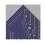# Summation Series to Product Series Part 1.

Here's something interesting I found out last year. I don't know if its been done before but I strongly suspect it has.

Consider a summation series of form $S(n) = \sum_{k = 1}^{k = n}\frac{f(k)}{k!}$ such that $f(1) = 1$.

Now let's do something unusual and create the following sequence starting with the term $a_2 = 2$ and then $a_{k+1} = (k + 1)(a_k + f(k))$ for all $k > 2$.

Finally we'll focus our attention on the following product series $P(n) = \prod_{k=2}^{k=n} ( 1 + \frac{f(k)}{a_k})$ for all $n > 2$.

We'll show that $S(n) = P(n)$ for all $n > 2$.

Proof : By induction on n. We'll start with the base case. Base Case: $n = 2$

We have $P(2) = 1 + \frac{f(2)}{a_2} = 1 + \frac{f(2)}{2} =\frac{f(1)}{1} + \frac{f(2)}{2} = S(2)$ as required.

Induction Step

This part is a quite long, so we'll break it down into 3 parts.

Part 1 : Re-arranging the Factors of the Product Series

We can write each factor from our product series as \begin{aligned} 1 + \frac{f(k)}{a_k} &= \frac{a_k}{a_k} + \frac{f(k)}{a_k} \\&= \frac{a_k + f(k)}{a_k} \\&= \frac{a_{k+1}}{(k+1)a_k} \end{aligned} by using the definition of our sequence.

Part 2 : Re-writing our Product Series

Now we have that \begin{aligned} \prod_{k = 2}^{k = n} (1 + \frac{f(k)}{a_k}) &= \frac{a_{3}}{(3)a_2} \times \frac{a_{4}}{(4)a_3} \times ...\times \frac{a_{n+1}}{(n+1)a_n} \\ &= \frac{a_{n+1}}{a_2 \times 3 \times 4 \times ... \times n \times (n+1)} \\&=\frac{a_{n+1}}{(n+1)!} \end{aligned}

Part 3 : Final Step

Suppose $P(q) = S(q)$ for some natural number $q$.

Then\begin{aligned} P(q+1) &= P(q)(1 + \frac{f(q+1)}{a_{q+1}}) \\&= P(q) + \frac{f(q+1)}{a_{q+1}}\times\frac{a_{q+1}}{(q+1)!} \\&= P(q) + \frac{f(q+1)}{(q+1)!} \\&= T(q) + \frac{f(q+1)}{(q+1)!} = T(q+1) \end{aligned} as required.

End of Proof

We'll go through some of the consequences of this in the next note!Note by Roberto Nicolaides
6 years, 5 months ago

This discussion board is a place to discuss our Daily Challenges and the math and science related to those challenges. Explanations are more than just a solution — they should explain the steps and thinking strategies that you used to obtain the solution. Comments should further the discussion of math and science.

When posting on Brilliant:

• Use the emojis to react to an explanation, whether you're congratulating a job well done , or just really confused .
• Ask specific questions about the challenge or the steps in somebody's explanation. Well-posed questions can add a lot to the discussion, but posting "I don't understand!" doesn't help anyone.
• Try to contribute something new to the discussion, whether it is an extension, generalization or other idea related to the challenge.

MarkdownAppears as
*italics* or _italics_ italics
**bold** or __bold__ bold
- bulleted- list
• bulleted
• list
1. numbered2. list
1. numbered
2. list
Note: you must add a full line of space before and after lists for them to show up correctly
paragraph 1paragraph 2

paragraph 1

paragraph 2

[example link](https://brilliant.org)example link
> This is a quote
This is a quote
    # I indented these lines
# 4 spaces, and now they show
# up as a code block.

print "hello world"
# I indented these lines
# 4 spaces, and now they show
# up as a code block.

print "hello world"
MathAppears as
Remember to wrap math in $$ ... $$ or $ ... $ to ensure proper formatting.
2 \times 3 $2 \times 3$
2^{34} $2^{34}$
a_{i-1} $a_{i-1}$
\frac{2}{3} $\frac{2}{3}$
\sqrt{2} $\sqrt{2}$
\sum_{i=1}^3 $\sum_{i=1}^3$
\sin \theta $\sin \theta$
\boxed{123} $\boxed{123}$